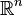This wiki is associated with Cognito Mentoring, an advising service for learners run by Jonah Sinick and Vipul Naik. The wiki is very much in beta, so you're likely to find many broken links and incomplete pages. Please be patient with us as we continue to improve our offerings.
Please connect with us to offer feedback on the wiki content.

This page describes major-specific or upper division parts of undergraduate mathematics, in contrast with lower division undergraduate mathematics (that includes calculus, multivariable calculus, linear algebra, and ordinary differential equations).

Major-specific material typically includes the following. In cases where the learning benefits and learning recommendations pages are missing, we haven't found enough topic-specific demand to investigate and find the best learning resources, but are open to doing so if people ask us questions about it.

Topic Brief description Learning benefits Learning recommendations
Undergraduate analysis A year-long sequence that includes some topological, measure-theoretic, and metric concepts associated with the real line, and an understanding of the real numbers and spaces likein that context. Some multivariable calculus and linear algebra may be covered here. Undergraduate analysis learning benefits Undergraduate analysis learning recommendations
Abstract algebra A year-long sequence that includes group theory, ring theory, and field theory. Some linear algebra may be covered here. Abstract algebra learning benefits Abstract algebra learning recommendations
Topology A single course covering point set topology + a glimpse of algebraic topology Topology learning benefits Topology learning recommendations
More analysis Courses in complex analysis, functional analysis, partial differential equations, analytic number theory, Fourier analysis -- --
More algebra Courses in ommutative algebra, algebraic geometry, algebraic number theory -- --
More geometry/topology Courses in differential geometry and Riemannian geometry. -- --
Set theory, logic Course in logic -- --
Discrete mathematics and combinatorics Courses in discrete mathematics and combinatorics (offerings are not standardized) -- --
Probability theory (close to statistics) See our probability and statistics learning recommendations
Other applied Numerical analysis, Linear programming and optimization -- --

Mathematics students may be required or encouraged to take courses in sister subjects such as statistics and computer science.